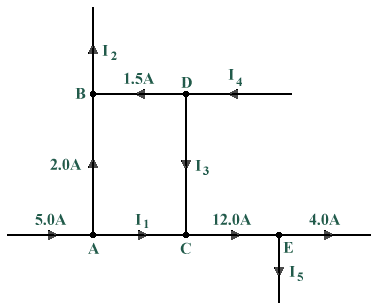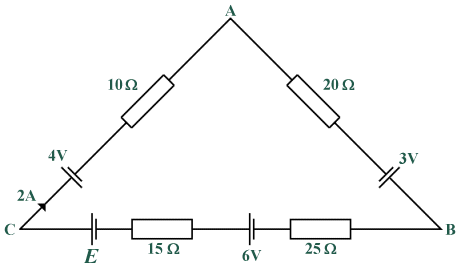# Answers to Problems - Kirchoff's Laws

1. In the diagram below, circuit nodes A through E each have one or more current flows into and out of them. You have been given some of the current values, and are required to apply Kirchhoff's current law to determine the value of the unknown currents I1 through I5.Find the unknown currents entering or leaving each node

The currents I1 to I5 are as follows:

I1  =  5.0 A  -  2.0 A  =  3.0 A

I2  =  1.5 A  +  2.0 A  =  3.5 A

I3  =  12.0 A  -  I1  =  12.0 A  -  3.0 A  =  9.0 A

I4  =  1.5 A  +  I3  =  1.5 A  +  9.0 A  =  10.5 A

I5  =  12.0 A  -  4.0 A  =  8.0 A

2. In the diagram below, four voltage sources are connected in series with four resistors. The value of one of the voltage sources, E, is unknown. Using what you know about Kirchhoff's voltage law, determine the voltage being supplied by E.Find the value of E

The value of E can be calculated as follows:

E  -  4 V  -  (2  ×  10) V  -  (2  ×  20) V  +  3 V  -  (2  ×  25) V  +  6 V  -  (2  ×  15) V  =  0

E  =  4 V  +  20 V  +  40 V  -  3 V  +  50 V  -  6 V  +  30 V

E  =  135 V